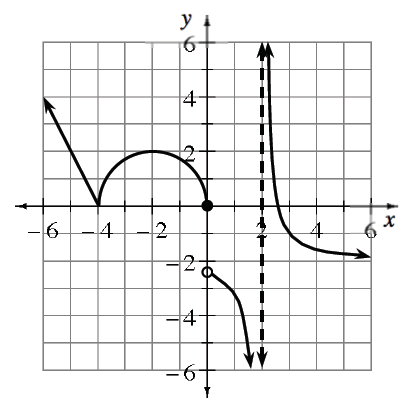### Home > APCALC > Chapter 3 > Lesson 3.1.1 > Problem3-13

3-13.

Is the function graphed at right continuous at the following values of $x$? If not, explain which conditions of continuity fail.

$x = −4, −2, 0,$ and $2$Look at your Math Notes for the three conditions of continuity.

Use the three conditions to test if the function is continuous at $x = −2, x = 0$ and $x = 2$.

Examine the graph at $x = −4$.

Condition 1:

$\lim \limits_{x\rightarrow -4^{-}}f(x)=0; \ \lim \limits_{x\rightarrow -4^{+}}f(x)=0$

$\lim \limits_{x\rightarrow -4^{-}}f(x)=\lim \limits_{x\rightarrow -4^{+}}f(x).$

$\text{Therefore }\lim \limits_{x\rightarrow -4}f(x)\text{ exists.}$

Condition 2:

$f\left(-4\right) = 0$.
Therefore $f\left(−4\right)$ exists.

Condition 3:

$\lim \limits_{x\rightarrow -4}f(x)=f(-4).$

The function is continuous at $x = −4$.